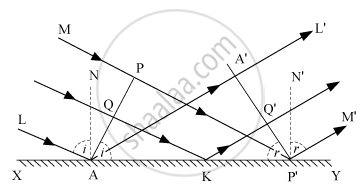# Answer the Following Question. Define the Term Wavefront. Using Huygen'S Wave Theory, Verify the Law of Reflection. - Physics

Define the term wavefront. Using Huygen's wave theory, verify the law of reflection.

#### Solution

A wavefront is defined as the locus of all points having the same phase at a given instant of time.

Derivation of Law of reflection:Consider any point Q on the incident wavefront PA.

When the disturbance from P on incident wavefront reaches point P', the disturbance from point Q reaches Q'.

If c is the velocity of light, then the time taken by light to go from point Q to Q'(via point K) is given by,

r = "QK"/c + "KQ'"/c    ...(i)

In right-angled ΔAQK,
∠QAK = ∠i

QK = AK sin i

In right-angled, ΔKQ'P'
∠Q'P'K = r

KQ' = KP' sin r

Substituting these values in equation (1),

t = ("AK" sin i)/(c) +("KP"' sin r)/(c)

t = ("AK" sin i + ("AP"' - "AK") sinr)/(c)   ...(∵ "KP"' = "AP"' - "AK"')

t = ("AP"' sinr + "AK"(sin i - sin r))/(c)   ....(ii)

The rays from different points on incident wavefront will take the same time to reach the corresponding points on the reflected wavefront, if ‘t’ given by equation (ii) is independent of AK.

∴ AK (sin i − sin r) = 0
sin i − sin r = 0
sin i = sin r
i = r

i.e., the angle of incidence is equal to the angle of reflection.
Also, the incident ray (LA or MP'), reflected ray (AA'L' or P'M'), and the normal (AN) − all lie in the same plane.

Concept: Huygens Principle
Is there an error in this question or solution?